Work, Energy, and Power - Lesson 1 - Basic Terminology and Concepts

# Mechanical Energy

In a previous part of Lesson 1, it was said that work is done upon an object whenever a force acts upon it to cause it to be displaced. Work involves a force acting upon an object to cause a displacement. In all instances in which work is done, there is an object that supplies the force in order to do the work. If a World Civilization book is lifted to the top shelf of a student locker, then the student supplies the force to do the work on the book. If a plow is displaced across a field, then some form of farm equipment (usually a tractor or a horse) supplies the force to do the work on the plow. If a pitcher winds up and accelerates a baseball towards home plate, then the pitcher supplies the force to do the work on the baseball. If a roller coaster car is displaced from ground level to the top of the first drop of a roller coaster ride, then a chain driven by a motor supplies the force to do the work on the car. If a barbell is displaced from ground level to a height above a weightlifter's head, then the weightlifter is supplying a force to do work on the barbell. In all instances, an object that possesses some form of energy supplies the force to do the work. In the instances described here, the objects doing the work (a student, a tractor, a pitcher, a motor/chain) possess chemical potential energy stored in food or fuel that is transformed into work. In the process of doing work, the object that is doing the work exchanges energy with the object upon which the work is done. When the work is done upon the object, that object gains energy. The energy acquired by the objects upon which work is done is known as mechanical energy.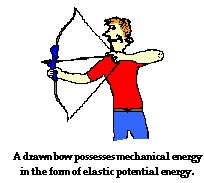Mechanical energy is the energy that is possessed by an object due to its motion or due to its position. Mechanical energy can be either kinetic energy (energy of motion) or potential energy (stored energy of position). Objects have mechanical energy if they are in motion and/or if they are at some position relative to a zero potential energy position (for example, a brick held at a vertical position above the ground or zero height position). A moving car possesses mechanical energy due to its motion (kinetic energy). A moving baseball possesses mechanical energy due to both its high speed (kinetic energy) and its vertical position above the ground (gravitational potential energy). A World Civilization book at rest on the top shelf of a locker possesses mechanical energy due to its vertical position above the ground (gravitational potential energy). A barbell lifted high above a weightlifter's head possesses mechanical energy due to its vertical position above the ground (gravitational potential energy). A drawn bow possesses mechanical energy due to its stretched position (elastic potential energy).

### Mechanical Energy as the Ability to Do WorkAn object that possesses mechanical energy is able to do work. In fact, mechanical energy is often defined as the ability to do work. Any object that possesses mechanical energy - whether it is in the form of potential energy or kinetic energy - is able to do work. That is, its mechanical energy enables that object to apply a force to another object in order to cause it to be displaced.

Numerous examples can be given of how an object with mechanical energy can harness that energy in order to apply a force to cause another object to be displaced. A classic example involves the massive wrecking ball of a demolition machine. The wrecking ball is a massive object that is swung backwards to a high position and allowed to swing forward into building structure or other object in order to demolish it. Upon hitting the structure, the wrecking ball applies a force to it in order to cause the wall of the structure to be displaced. The diagram below depicts the process by which the mechanical energy of a wrecking ball can be used to do work.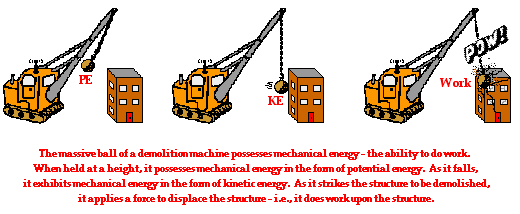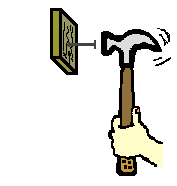A hammer is a tool that utilizes mechanical energy to do work. The mechanical energy of a hammer gives the hammer its ability to apply a force to a nail in order to cause it to be displaced. Because the hammer has mechanical energy (in the form of kinetic energy), it is able to do work on the nail. Mechanical energy is the ability to do work.Another example that illustrates how mechanical energy is the ability of an object to do work can be seen any evening at your local bowling alley. The mechanical energy of a bowling ball gives the ball the ability to apply a force to a bowling pin in order to cause it to be displaced. Because the massive ball has mechanical energy (in the form of kinetic energy), it is able to do work on the pin. Mechanical energy is the ability to do work.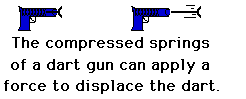A dart gun is still another example of how mechanical energy of an object can do work on another object. When a dart gun is loaded and the springs are compressed, it possesses mechanical energy. The mechanical energy of the compressed springs gives the springs the ability to apply a force to the dart in order to cause it to be displaced. Because of the springs have mechanical energy (in the form of elastic potential energy), it is able to do work on the dart. Mechanical energy is the ability to do work.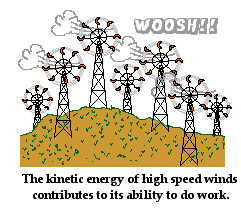A common scene in some parts of the countryside is a "wind farm." High-speed winds are used to do work on the blades of a turbine at the so-called wind farm. The mechanical energy of the moving air gives the air particles the ability to apply a force and cause a displacement of the blades. As the blades spin, their energy is subsequently converted into electrical energy (a non-mechanical form of energy) and supplied to homes and industries in order to run electrical appliances. Because the moving wind has mechanical energy (in the form of kinetic energy), it is able to do work on the blades. Once more, mechanical energy is the ability to do work.

### The Total Mechanical Energy

As already mentioned, the mechanical energy of an object can be the result of its motion (i.e., kinetic energy) and/or the result of its stored energy of position (i.e., potential energy). The total amount of mechanical energy is merely the sum of the potential energy and the kinetic energy. This sum is simply referred to as the total mechanical energy (abbreviated TME).

TME = PE + KE

As discussed earlier, there are two forms of potential energy discussed in our course - gravitational potential energy and elastic potential energy. Given this fact, the above equation can be rewritten:

TME = PEgrav + PEspring + KE

The diagram below depicts the motion of Lee Ben Fardest (esteemed American ski jumper) as he glides down the hill and makes one of his record-setting jumps.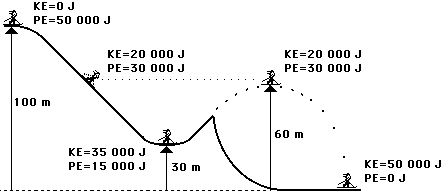The total mechanical energy of Lee Ben Fardest is the sum of the potential and kinetic energies. The two forms of energy sum up to 50 000 Joules. Notice also that the total mechanical energy of Lee Ben Fardest is a constant value throughout his motion. There are conditions under which the total mechanical energy will be a constant value and conditions under which it will be a changing value. This is the subject of Lesson 2 - the work-energy relationship. For now, merely remember that total mechanical energy is the energy possessed by an object due to either its motion or its stored energy of position. The total amount of mechanical energy is merely the sum of these two forms of energy. And finally, an object with mechanical energy is able to do work on another object.

Next Section: